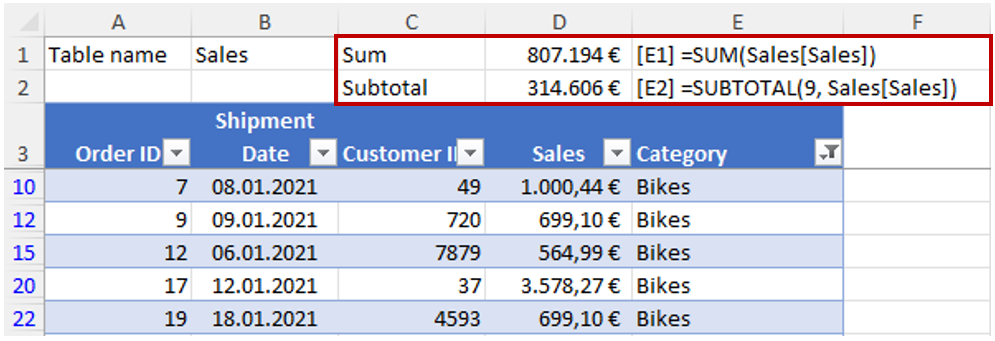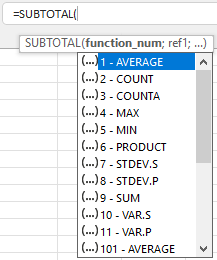# Calculate sums and subtotals

Use both SUMs and SUBTOTALs to help understand your data!• Place SUMs for key data columns such as sales values above your data where you can easily see them and not below your data, which forces you to scroll down – that is inefficient, especially if you have long lists.

• Also calculate SUBTOTALs. SUBTOTAL is a very useful and flexible function which shows you the sum of your filtered data, very handy when checking or analysing your data.The SUBTOTAL function can give various results depending upon the number you enter in brackets before the data range. Most commonly you want the sum, which requires the number 9. Other possibilities are average, count, maximum and minimum. As soon as you type SUBTOTAL( you get a drop-down list of all the options.

I recommend that you calculate both SUM and SUBTOTALS for key data columns in two separate rows, as shown in the screenshot. The SUM result showing the total of all amounts in the column is then always visible and can be linked onwards in the workbook (if necessary) and the SUBTOTAL can be used for filtering and data analysis. If no filters are set, the two formulas should give the same result.

Note: In the example in the screenshot I have used a table but both SUM and SUBTOTAL functions also work with data which is not in a table. In this case, simply enter the cell references as your data range.